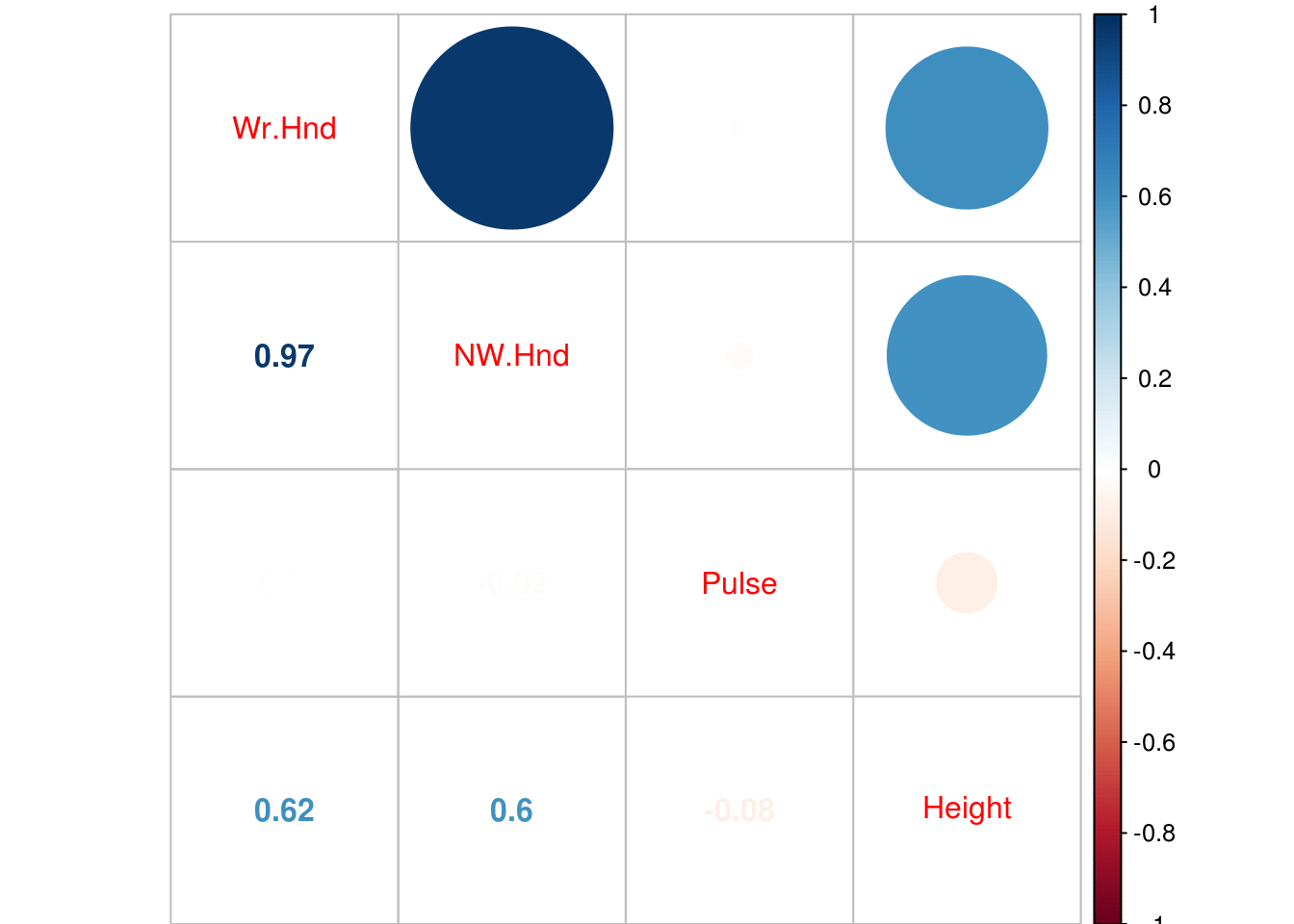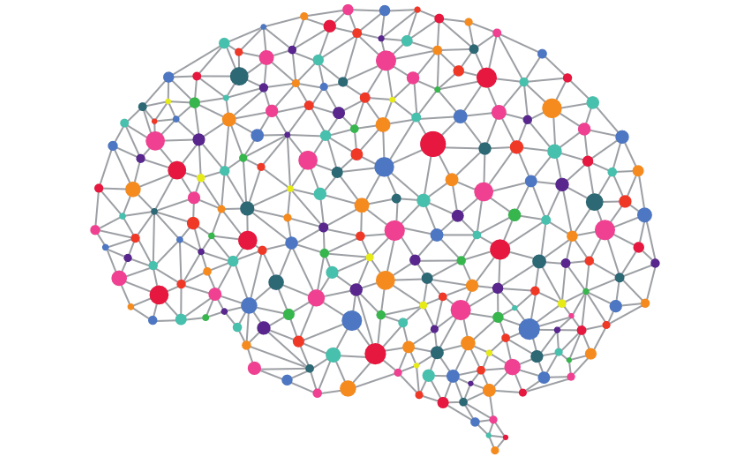# Logistic Regression in R

In this post, we will conduct a logistic regression analysis. Logistic regression is used when you want to predict a categorical dependent variableusing continuous or categorical dependent variables. In our example, we want to predict Sex (male or female) when using several continuous variables from the “survey” dataset in the “MASS” package.

``library(MASS);library(bestglm);library(reshape2);library(corrplot)``
``````data(survey)
?MASS::survey #explains the variables in the study``````

The first thing we need to do is remove the independent factor variables from our dataset. The reason for this is that the function that we will use for the cross-validation does not accept factors. We will first use the “str” function to identify factor variables and then remove them from the dataset. We also need to remove in examples that are missing data so we use the “na.omit” function for this. Below is the code

``````survey\$Clap<-NULL
survey\$W.Hnd<-NULL
survey\$Fold<-NULL
survey\$Exer<-NULL
survey\$Smoke<-NULL
survey\$M.I<-NULL
survey<-na.omit(survey)``````

We now need to check for collinearity using the “corrplot.mixed” function form the “corrplot” package.

``````pc<-cor(survey[,2:5])
corrplot.mixed(pc)
corrplot.mixed(pc)``````We have an extreme correlation between “We.Hnd” and “NW.Hnd” this makes sense because people’s hands are normally the same size. Since this blog post is a demonstration of logistic regression we will not worry about this too much.

We now need to divide our dataset into a train and a test set. We set the seed for. First, we need to make a variable that we call “ind” that is randomly assigned 70% of the number of rows of survey 1 and 30% 2. We then subset the “train” dataset by taking all rows that are 1’s based on the “ind” variable and we create the “test” dataset for all the rows that line up with 2 in the “ind” variable. This means our data split is 70% train and 30% test. Below is the code

``````set.seed(123)
ind<-sample(2,nrow(survey),replace=T,prob = c(0.7,0.3))
train<-survey[ind==1,]
test<-survey[ind==2,]``````

We now make our model. We use the “glm” function for logistic regression. We set the family argument to “binomial”. Next, we look at the results as well as the odds ratios.

``````fit<-glm(Sex~.,family=binomial,train)
summary(fit)``````
``````##
## Call:
## glm(formula = Sex ~ ., family = binomial, data = train)
##
## Deviance Residuals:
##     Min       1Q   Median       3Q      Max
## -1.9875  -0.5466  -0.1395   0.3834   3.4443
##
## Coefficients:
##              Estimate Std. Error z value Pr(>|z|)
## (Intercept) -46.42175    8.74961  -5.306 1.12e-07 ***
## Wr.Hnd       -0.43499    0.66357  -0.656    0.512
## NW.Hnd        1.05633    0.70034   1.508    0.131
## Pulse        -0.02406    0.02356  -1.021    0.307
## Height        0.21062    0.05208   4.044 5.26e-05 ***
## Age           0.00894    0.05368   0.167    0.868
## ---
## Signif. codes:  0 '***' 0.001 '**' 0.01 '*' 0.05 '.' 0.1 ' ' 1
##
## (Dispersion parameter for binomial family taken to be 1)
##
##     Null deviance: 169.14  on 122  degrees of freedom
## Residual deviance:  81.15  on 117  degrees of freedom
## AIC: 93.15
##
## Number of Fisher Scoring iterations: 6``````
``exp(coef(fit))``
``````##  (Intercept)       Wr.Hnd       NW.Hnd        Pulse       Height
## 6.907034e-21 6.472741e-01 2.875803e+00 9.762315e-01 1.234447e+00
##          Age
## 1.008980e+00``````

The results indicate that only height is useful in predicting if someone is a male or female. The second piece of code shares the odds ratios. The odds ratio tell how a one unit increase in the independent variable leads to an increase in the odds of being male in our model. For example, for every one unit increase in height there is a 1.23 increase in the odds of a particular example being male.

We now need to see how well our model does on the train and test dataset. We first capture the probabilities and save them to the train dataset as “probs”. Next we create a “predict” variable and place the string “Female” in the same number of rows as are in the “train” dataset. Then we rewrite the “predict” variable by changing any example that has a probability above 0.5 as “Male”. Then we make a table of our results to see the number correct, false positives/negatives. Lastly, we calculate the accuracy rate. Below is the code.

``````train\$probs<-predict(fit, type = 'response')
train\$predict<-rep('Female',123)
train\$predict[train\$probs>0.5]<-"Male"
table(train\$predict,train\$Sex)``````
``````##
##          Female Male
##   Female     61    7
##   Male        7   48``````
``mean(train\$predict==train\$Sex)``
``##  0.8861789``

Despite the weaknesses of the model with so many insignificant variables it is surprisingly accurate at 88.6%. Let’s see how well we do on the “test” dataset.

``````test\$prob<-predict(fit,newdata = test, type = 'response')
test\$predict<-rep('Female',46)
test\$predict[test\$prob>0.5]<-"Male"
table(test\$predict,test\$Sex)``````
``````##
##          Female Male
##   Female     17    3
##   Male        0   26``````
``mean(test\$predict==test\$Sex)``
``##  0.9347826``

As you can see, we do even better on the test set with an accuracy of 93.4%. Our model is looking pretty good and height is an excellent predictor of sex which makes complete sense. However, in the next post we will use cross-validation and the ROC plot to further assess the quality of it.# Classification Rules in Machine Learning

Classification rules represent knowledge in an if-else format. These types of rules involve the terms antecedent and consequent. The antecedent is the before and consequent is after. For example, I may have the following rule.

If the students studies 5 hours a week then they will pass the class with an A

This simple rule can be broken down into the following antecedent and consequent.

• Antecedent–If the student studies 5 hours a week
• Consequent-then they will pass the class with an A

The antecedent determines if the consequent takes place. For example, thestudent must study 5 hours a week to get an A. This is the rule in this particular context.

This post will further explain the characteristics and traits of classification rules.

Classification Rules and Decision Trees

Classification rules are developed on current data to make decisions about future actions. They are highly similar to the more common decision trees. The primary difference is that decision trees involve a complex step-by-step process to make a decision.

Classification rules are stand-alone rules that are abstracted from a process. To appreciate a classification rule you do not need to be familiar with the process that created it. While with decision trees you do need to be familiar with the process that generated the decision.

One catch with classification rules in machine learning is that the majority of the variables need to be nominal in nature. As such, classification rules are not as useful for large amounts of numeric variables. This is not a problem with decision trees.

The Algorithm

Classification rules use algorithms that employ a separate and conquer heuristic. What this means is that the algorithm will try to separate the data into smaller and smaller subset by generating enough rules to make homogeneous subsets. The goal is always to separate the examples in the data set into subgroups that have similar characteristics.

Common algorithms used in classification rules include the One Rule Algorithm and the RIPPER Algorithm. The One Rule Algorithm analyzes data and generates one all-encompassing rule. This algorithm works by finding the single rule that contains the less amount of error. Despite its simplicity, it is surprisingly accurate.

The RIPPER algorithm grows as many rules as possible. When a rule begins to become so complex that in no longer helps to purify the various groups the rule is pruned or the part of the rule that is not beneficial is removed. This process of growing and pruning rules is continued until there is no further benefit.

RIPPER algorithm rules are more complex than One Rule Algorithm. This allows for the development of complex models. The drawback is that the rules can become too complex to make practical sense.

Conclusion

Classification rules are a useful way to develop clear principles as found in the data. The advantage of such an approach is simplicity. However, numeric data is harder to use when trying to develop such rules.